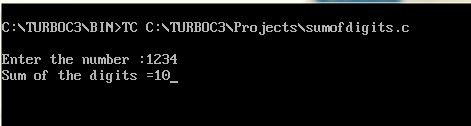UncleCoder.com

UncleCoder.com

Free programming examples and instructions

# C Program to find sum of digits

## C Program for how to find sum of digits

by Krishna

Posted on 27 Jul 2018 Category: C Views: 1216

C Program to find sum of digits

In this program, we will see a C program to calculate the sum of digits of a number. In this program, we will use modulus operator (%) to extract individual digits of a number and keep on adding them. This program can also be done withput using % modulus operator.

``````#include <stdio.h>
int main()
{
int n, t, sum = 0, remainder;
printf("Enter the number :");
scanf("%d", &n);
t = n;
while (t != 0)
{
remainder = t % 10;
sum = sum + remainder;
t = t / 10;
}
printf("Sum of digits = %d\n", sum);
return 0;
}``````

OUTPUT